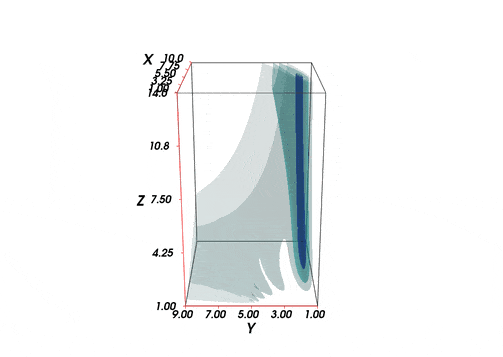This figure is the Fig2 of my research work and I let it rotated.

This time I try o plot the 3d animated distribution via python package Mayavi

Different from the animated operation via using the Matplotlib, This 3d data visualization is more powerful.

I only upload the figure this time and I will write a complete note latterly.

The data are calculated via parameter sweep and store with txt format. We need load it

We need transpose the matrix since I need kappa to be the z axi

Then we can plot the 3d data. The static plot could be plotted first

then we should define a animated function. Since in this case we only need update the view rather than the data. So the code is a little bit diffeent

The view’s azimuth angle will update each frame. If we want to handle the data, we should use the “mlab.source” to update the arguement as is shown in the offical example

We finally export the gif file and I learned this from this Blog

The figure is a little big and we can compress this gif file in the online web

The following is the figure we obtain